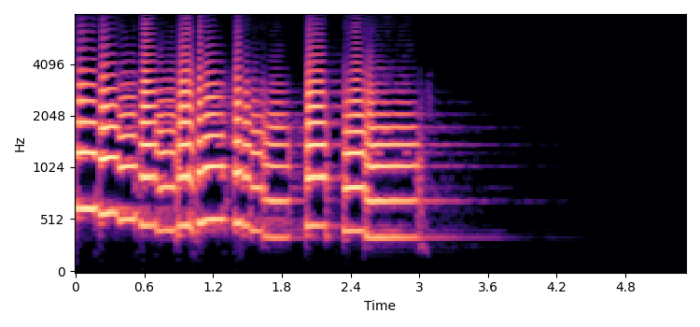# How to save a Librosa spectrogram plot as a specific sized image?

Librosa is a Python package that helps to analyse audio and music files. This package also helps to create music retrieval information systems. In this article, we will see how to save a Librosa spectrogram plot as an image of specific size.

## Steps

• Set the figure size and adjust the padding between and around the subplots..

• Create a figure and a set of subplots.

• Initialize three different variables, hl, hi, wi, to store samples per time in the spectrogram, height and width of the images.

• Create a window, i.e., a list for audio time series..

• Compute a mel-scaled spectrogram, using melspectrogram() with window and step 3 data.

• Convert the power spectrogram (amplitude squared) to decibel (dB) units, using power_to_db() method..

• Display the spectrogram as img (we can save it here).

• Save the img using savefig().

• Display the image using plt.show() method.

## Example

import numpy as np
import matplotlib.pyplot as plt
import librosa.display

plt.rcParams["figure.figsize"] = [7.50, 3.50]
plt.rcParams["figure.autolayout"] = True

fig, ax = plt.subplots()

hl = 512 # number of samples per time-step in spectrogram
hi = 128 # Height of image
wi = 384 # Width of image

window = y[0:wi*hl]

S = librosa.feature.melspectrogram(y=window, sr=sr, n_mels=hi, fmax=8000,
hop_length=hl)
S_dB = librosa.power_to_db(S, ref=np.max)
img = librosa.display.specshow(S_dB, x_axis='time', y_axis='mel', sr=sr, fmax=8000, ax=ax)

plt.savefig("out.png")
plt.show()

## OutputUpdated on: 15-Feb-2022

4K+ Views